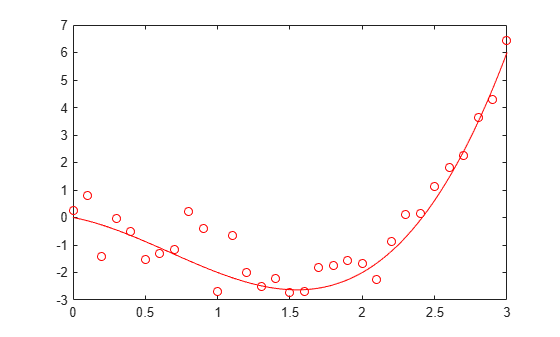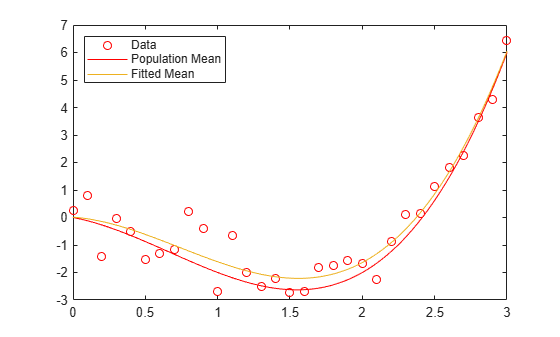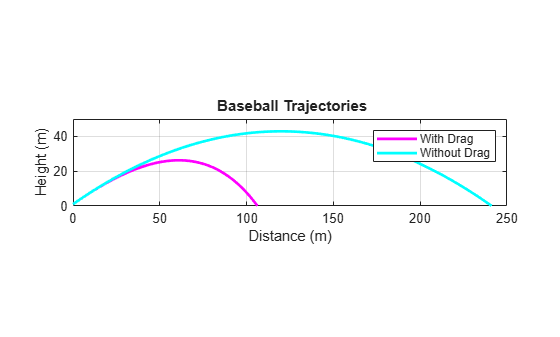refcurve

Syntax

refcurve(p)
refcurve
refcurve(ax,p)
hcurve = refcurve(...)

Description

refcurve(p) adds a polynomial reference curve with coefficients p to the current axes. If p is a vector with n+1 elements, the curve is:

y = p(1)*x^n + p(2)*x^(n-1) + ... + p(n)*x + p(n+1)

refcurve with no input arguments adds a line along the x axis.

refcurve(ax,p) uses the plot axes specified in ax, an Axes object. For more information, see axes.

hcurve = refcurve(...) returns the handle hcurve to the curve using any of the input argument combinations in the previous syntaxes.

Examples

collapse all

Generate data with a polynomial trend.

p = [1 -2 -1 0];
t = 0:0.1:3;
rng default  % For reproducibility
y = polyval(p,t) + 0.5*randn(size(t));

Plot data and add the population mean function using refcurve .

plot(t,y,'ro')
h = refcurve(p);
h.Color = 'r';Also add the fitted mean function.

q = polyfit(t,y,3);
refcurve(q)
legend('Data','Population Mean','Fitted Mean',...
'Location','NW')Introduce the relevant physical constants.

M = 0.145;      % Mass (kg)
R = 0.0366;     % Radius (m)
A = pi*R^2;     % Area (m^2)
rho = 1.2;      % Density of air (kg/m^3)
C = 0.5;        % Drag coefficient
D = rho*C*A/2;  % Drag proportional to the square of the speed
g = 9.8;        % Acceleration due to gravity (m/s^2)

Simulate the trajectory with drag proportional to the square of the speed, assuming constant acceleration in each time interval.

dt = 1e-2;      % Simulation time interval (s)
r0 = [0 1];     % Initial position (m)
s0 = 50;        % Initial speed (m/s)
alpha0 = 35;    % Initial angle (deg)
v0 = s0*[cosd(alpha0) sind(alpha0)]; % Initial velocity (m/s)

r = r0;
v = v0;
trajectory = r0;
while r(2) > 0
a = [0 -g] - (D/M)*norm(v)*v;
v = v + a*dt;
r = r + v*dt + (1/2)*a*(dt^2);
trajectory = [trajectory;r];
end

Plot trajectory and use refcurve to add the drag-free parabolic trajectory (found analytically) to the plot of trajectory.

figure
plot(trajectory(:,1),trajectory(:,2),'m','LineWidth',2)
xlim([0,250])
h = refcurve([-g/(2*v0(1)^2),...
(g*r0(1)/v0(1)^2) + (v0(2)/v0(1)),...
(-g*r0(1)^2/(2*v0(1)^2)) - (v0(2)*r0(1)/v0(1)) + r0(2)]);
h.Color = 'c';
h.LineWidth = 2;
axis equal
ylim([0,50])
grid on
xlabel('Distance (m)')
ylabel('Height (m)')
title('{\bf Baseball Trajectories}')
legend('With Drag','Without Drag')